# Test: Electrical & Electronic Measurements- 2

## 20 Questions MCQ Test GATE Electrical Engineering (EE) 2023 Mock Test Series | Test: Electrical & Electronic Measurements- 2

Description
Attempt Test: Electrical & Electronic Measurements- 2 | 20 questions in 60 minutes | Mock test for GATE preparation | Free important questions MCQ to study GATE Electrical Engineering (EE) 2023 Mock Test Series for GATE Exam | Download free PDF with solutions
QUESTION: 1

### Two meters X and Y require 40 mA and 50 mA, respectively, to give full-scale deflection, then

Solution:

Refer static sensitivity.

QUESTION: 2

### What is the correct sequence of the following types of ammeters and voltmeters with increasing accuracy? 1. Moving iron 2. Moving-coil permanent magnet 3. Induction Select the correct answer using the codes given below:

Solution:

Induction principle is more generally used for watt-hour meters than for ammeters and voltmeters owing to their comparatively high cost, and inaccuracy of induction instruments of the latter types.

QUESTION: 3

### The total current I = I1 + I2 in a circuit is measured as I1 = 150 ± 1 A, I2 = 250 ± 2 A, where the limits of error are given as standard deviations. I is measured as

Solution: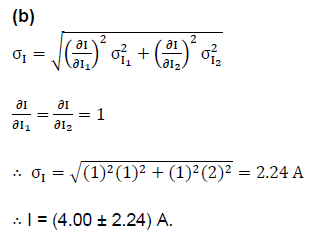QUESTION: 4

For the bridge shown in the given figure,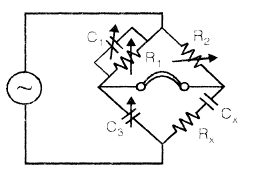Solution:

(a)
Refer Schering Bridge

QUESTION: 5

Consider the following statements associated with moving iron instruments:

1. These can be used in d.c. as well a.c. circuits

2. The scale is non-uniform

3. The moving iron is placed in a field of a permanent magnet.

Which of these statements are correct?

Solution:

(b)
Moving iron is not placed in a field of a permanent magnet. Moving iron instrument employs an electromagnet and the iron vane moves in such a way so as to increase the flux of the electromagnet.

QUESTION: 6

In an oscilloscope, two Lissajous figure (X) and (Y) are observed. This indicates that ratio of vertical input signal frequency to that of horizontal input frequency are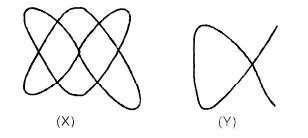Solution: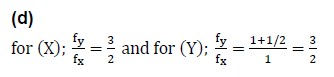QUESTION: 7

A spring controlled moving iron voltmeter draws a current of 1 mA for full scale value of 100 V. If it draws a current of 0.5 mA, the meter reading is

Solution: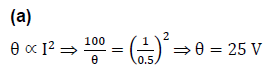QUESTION: 8

In the measurement of power on balanced load by two Wattmeter method in a 3-phase circuit, the readings of the Wattmeters are 3 kW and 1 kW respectively, the latter being obtained after reversing the connections to the current coil. The power factor of the load is

Solution:

(a)
When p.f. < 0.5 one of the wattmeter reads negative power; which is read after reversing the connections to the current coil or pressure coil. Also by formula: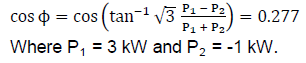QUESTION: 9

Two types of connections of Wattmeter pressure coil are shown in the figures.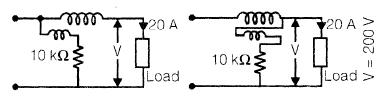The value of the Wattmeter current coil resistance r, which makes the connection errors the same in the two cases is

Solution: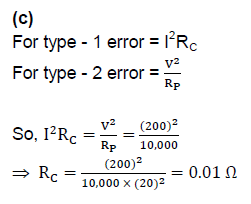QUESTION: 10

In calibration of a dynamometer Wattmeter by potentiometer, phantom loading arrangement is used because

Solution:

(b)

QUESTION: 11

The accuracy of Kelvin's double bridge for the measurement of low resistance is high because the bridge

Solution:

(a)
Kelvin Bridge overcomes the difficulties that arise in a Wheatstone bridge on account of the resistance of the leads and the contact resistances while measuring low valued resistors. The Kelvin double bridge incorporates the idea of a second set of ratio arms, hence the name double bridge and the use of four terminal resistors for the low resistance arms. The second set of ratio arms eliminates the effect of connecting lead resistance.

QUESTION: 12

An energy-meter having a meter constant of 1200 rev per kWH is found to make 5 revolutions in 75 s. The load power is

Solution:

(c)
Number of revolutions = K × P × t
∴ 5=1200×P×(75/3600)

⇒ P = 200 W

QUESTION: 13

The difference between the Indicated value and the true value of a quantity is

Solution:
QUESTION: 14

A Wien-bridge is used to measure the frequency of the input signal. However, the input signal has 10% third harmonic distortion. Specifically the signal is 2 sin 400 πt + 0.2 sin 1200 πt (with t insec). With this input the balance will

Solution:
QUESTION: 15

In a Q-meter measurement to determine the self-capacitance of a coil, the first resonance occurred at f1 with C1 = 300 pF. The second resonance occurred at f2 = 2f1 with C2 = 60 pF. The selfcapacitance of coil works out to be

Solution:

Self-capacitance Cd=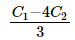QUESTION: 16

A multimeter is used for the measurement of the following

1. Both a.c. and d.c. voltage

2. Both a.c. and d.c. current

3. Resistance

4. Frequency

5. Power

Select the correct answer using the codes given below:

Solution:
QUESTION: 17

Three d.c. voltmeters are connected in series across a 120 V d.c. supply. The voltmeters are specified as follows: Voltmeter A : 100 V, 5 mA Voltmeter

B : 100 V, 250 ohms/V Voltmeter

C : 10 mA, 15,000 ohms

The voltages read by the meters A, B and C are respectively

Solution: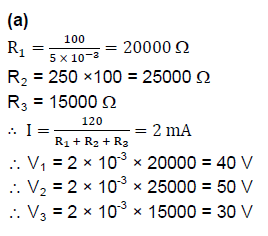QUESTION: 18

The pressure coil of an induction type energy meter is

Solution:
QUESTION: 19

The reflecting mirror mounted on the moving coil of a vibration galvanometer is replaced by a bigger size mirror. This will result in

Solution:

(a)
Bigger size mirror results in increased inertia constant J.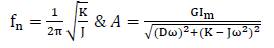as J increases both fn & A decrease.

QUESTION: 20

A screen pattern oscillogram, shown in the given figure is obtained when a sine-wave signal of unknown frequency is connected to the vertical input terminals and at the same time, a 600 Hz sinewave voltage is connected to the horizontal input terminals of an oscilloscope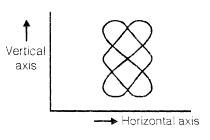What is the value of unknown frequency?

Solution:

Refer Lissajous Pattern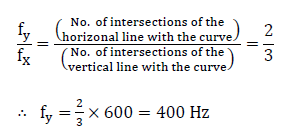Use Code STAYHOME200 and get INR 200 additional OFF Use Coupon Code